## Example Questions

← Previous 1

### Example Question #1 : Algebraic Fractions

A train travels at a constant rate ofmeters per second. How many kilometers does it travel inminutes?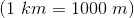Explanation:

Set up the conversions as fractions and solve: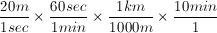### Example Question #2 : How To Simplify A Fraction

Which quantity is greater?

Quantity A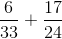Quantity B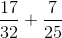Quantity A is greater.

Quantity B is greater.

The two quantities are equal.

The relationship cannot be determined from the information given.

Quantity A is greater.

Explanation:

This can be solved using 2 methods.

The most time-efficient solution would recognize that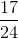is the largest value and nearly equals the sum the other fraction by itself.

The more time consuming method would be to convert each fraction to decimal form and calculate the sum of each quantity.

Quantity A: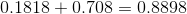Quantity B:### Example Question #2 : Algebraic Fractions

Simplify.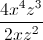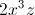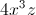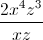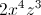Can't be simplifiedExplanation:

To simplify exponents which are being divided, subtract the exponents on the bottom from exponents on the top.  Remember that only exponents with the same bases can be simplified

### Example Question #3 : Algebraic Fractions

Simplify: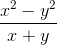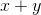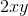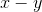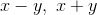Explanation:

x2 – y2 can be also expressed as (x + y)(x – y).

Therefore, the fraction now can be re-written as (x + y)(x – y)/(x + y).

This simplifies to (x – y).

### Example Question #4 : Algebraic Fractions

Simplify: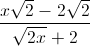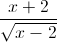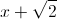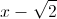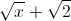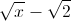Explanation:

Notice that the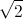term appears frequently. Let's try to factor that out: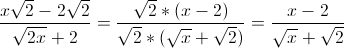Now multiply both the numerator and denominator by the conjugate of the denominator: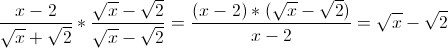### Example Question #6 : How To Simplify A Fraction

Simplify:

(2x + 4)/(x + 2)

2x + 2

x + 2

2

x + 1

x + 4

2

Explanation:

(2x + 4)/(x + 2)

To simplify you must first factor the top polynomial to 2(x + 2). You may then eliminate the identical (x + 2) from the top and bottom leaving 2.

### Example Question #3 : Algebraic Fractions

Simplify the following expression: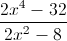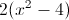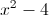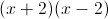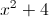Explanation:

Factor both the numerator and the denominator: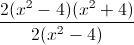After reducing the fraction, all that remains is: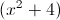### Example Question #1 : Algebraic Fractions

Simplify: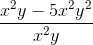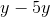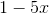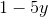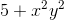Explanation:

With this problem the first thing to do is cancel out variables. The x2 can all be divided by each other because they are present in each system. The equation will now look like this: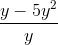Now we can see that the equation can all be divided by y, leaving the answer to be:### Example Question #9 : How To Simplify A Fraction

Simplify the given fraction: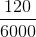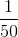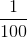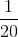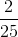Explanation:

120 goes into 6000 evenly 50 times, so we get 1/50 as our simplified fraction.

### Example Question #1311 : Psat Mathematics

Simplify the given fraction: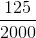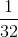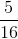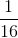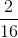Explanation:

125 goes into 2000 evenly 16 times. 1/16 is the fraction in its simplest form.

← Previous 1

Tired of practice problems?

Try live online GRE prep today.